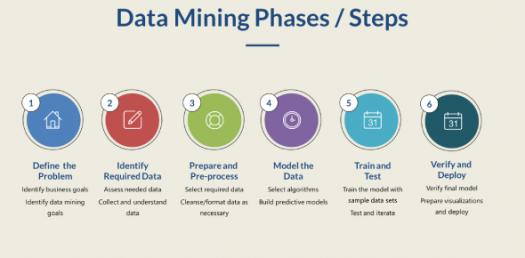# Data Mining Quiz Data Mining Course

10 Questions | Total Attempts: 3271SettingsData is an important aspect of information gathering for assessment and thus data mining is essential. Through the quiz below you will be able to find out more about data mining and how to go about it. Take it up.

Related Topics
• 1.
• A.

Supervised learning

• B.

Unsupervised learning

• C.

Reinforcement learning

• 2.
Task of inferring a model from labeled training data is called
• A.

Unsupervised learning

• B.

Supervised learning

• C.

Reinforcement learning

• 3.
• A.

Supervised learning

• B.

Data extraction

• C.

Seriation

• D.

Unsupervised learning

• 4.
Self-organizing maps is an example of ...
• A.

Unsupervised learning

• B.

Supervised learning

• C.

Reinforcement learning

• D.

Missing data imputation

• 5.
You are given data about seismic activity in Japan, and you want to predict a magnitude of the next earthquake, this is in an example of ...
• A.

Supervised learning

• B.

Unsupervised learning

• C.

Seriation

• D.

Dimensionality reduction

• 6.
Assume you want to perform supervised learning and to predict number of newborns according to size of storks' population (http://www.brixtonhealth.com/storksBabies.pdf), it is an example of ...
• A.

Classification

• B.

Regression

• C.

Clustering

• D.

Structural equation modeling

• 7.
Discriminating between spam and ham e-mails  is a classification task, true or false?
• A.

True

• B.

False

• 8.
In the example of predicting number of babies based on storks' population size, number of babies is ...
• A.

Outcome

• B.

Feature

• C.

Attribute

• D.

Observation

• 9.
It may be better to avoid the metric of ROC curve as it can suffer from accuracy paradox.
• A.

True

• B.

False